#### Z - TEST EXAMPLES AND CALCULATOR

“Facts are stubborn things, but statistics are pliable.” Mark Twain

Z - Test

A Z-test is a type of hypothesis test. Hypothesis testing is just a way for you to figure out if results from a test are valid or repeatable. For example, if someone said they had found a new drug that cures cancer, you would want to be sure it was probably true. A hypothesis test will tell you if it’s probably true, or probably not true. A Z test, is used when your data is approximately normally distributed.

A z-test is used for testing the mean of a population versus a standard, or comparing the means of two populations, with large (n ≥ 30) samples whether you know the population standard deviation or not. It is also used for testing the proportion of some characteristic versus a standard proportion, or comparing the proportions of two populations.

Example:Comparing the average engineering salaries of men versus women.

Example: Comparing the fraction defectives from 2 production lines.

Conditions:

You would use a Z test if:
Your sample size is greater than 30. Otherwise, use a t test.
Data points should be independent from each other. In other words, one data point isn’t related or doesn’t affect another data point.
Your data should be normally distributed. However, for large sample sizes (over 30) this doesn’t always matter.
Your data should be randomly selected from a population, where each item has an equal chance of being selected.
Sample sizes should be equal if at all possible.

Sample question for two proportion Z test:

Let’s say you’re testing two flu drugs A and B. Drug A works on 41 people out of a sample of 195. Drug B works on 351 people in a sample of 605. Are the two drugs comparable? Use a 5% alpha level.

Step 1: From the problem, we have: k2 = 41; n2 = 195; k1 = 351 and n1 = 605. Find the two proportions:
P2-hat = k1/n1 = 41/195 = 0.21
P1-hat = k2/n2 = 351/605 = 0.58
Hint: Choose index p1-hat for the bigger number
Find the overall sample proportion:
p-hat = (k1 + k2)/(n1 + n2) = (41 + 351) / (195 + 605) = 0.49

Step 2: Insert the numbers from Step 1 into the following test statistic formula: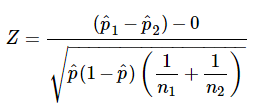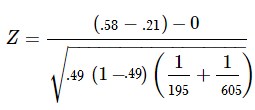Solving the formula, we get:
Z = 8.99
We need to find out if the z-score falls into the “rejection region."

Step 3: Find the z-score associated with α/2. We use the following table of known values: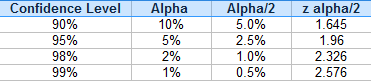The z-score associated with α/2 = 0.05/ 2 = 0.025 is 1.96
Because Z = 8.99 is larger than 1.96, we can reject the null hypothesis.

HYPOTHESIS TEST FOR THE DIFFERENCE OF TWO POPULATION PROPORTIONS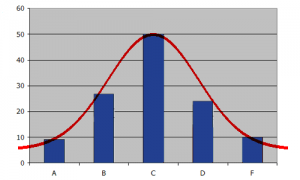Enter given data:

Input k1:
Input n1:
Input k2:
Input n2:
Input α :

Z alpha: Z test:

Null Hypothesis:

Sample question for one sample Z test:

A principle at a certain school claims that his students are above average intelligence. A random sample of 30 students IQ scores have a mean score of 112. Is there sufficient evidence to support the principle's claim? The mean population IQ is 100 with a standard deviation of 15. IQ scores are normally distributed.

Step 1: From the problem, we have: x-bar = 112; muy = 100; sigma = 15 ; n = 30 and alpha = 5% (default value). Use the following formula to find z test: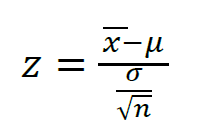z = (112- 100)/(15/sqrt(30))
z = 4.38

Step 2: Use the default value for alpha 5%, right tail test, and z table, we can find z alpha = 1.645
We see that the z-score falls into the “right tail 5% region."
Because Z = 4.38 is larger than 1.645, we can reject the null hypothesis and accept the principle's claim.

HYPOTHESIS TEST FOR ONE SAMPLE Z TEST

Enter given data:

Input Xbar:
Input muy:
Input n :
Input sigma:
Input alpha:

Z alpha: Z test:

Null Hypothesis: